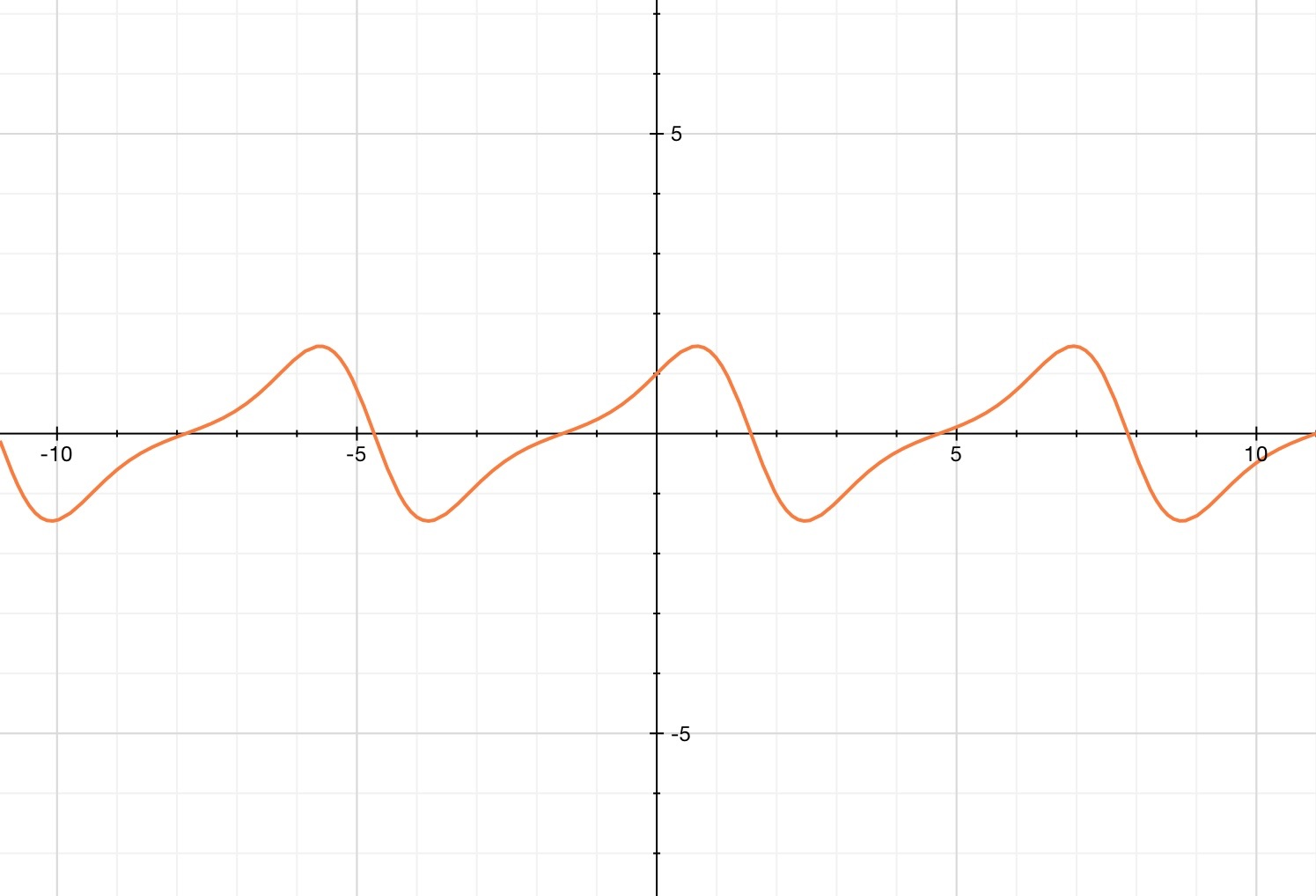# The Greatest Sin

Calculus Level 4$f(x)=e^{\sin(x)}\cos(x)$

Let $f(x)$ denote the function of the expression above. If the greatest possible value of $f(x)$ can be represented by the form $\left(\dfrac{\sqrt{c}-b}{a}\right)^{\large\frac{1}{a}} \cdot e^{\stackrel{\large\frac{\sqrt{c}-b}{a}}{}}$

Where $a,b,c$ are constants and $e\approx 2.7183...$ (Euler's constant) and $a,b,c$ are integers. Find $a+b+c$.

×